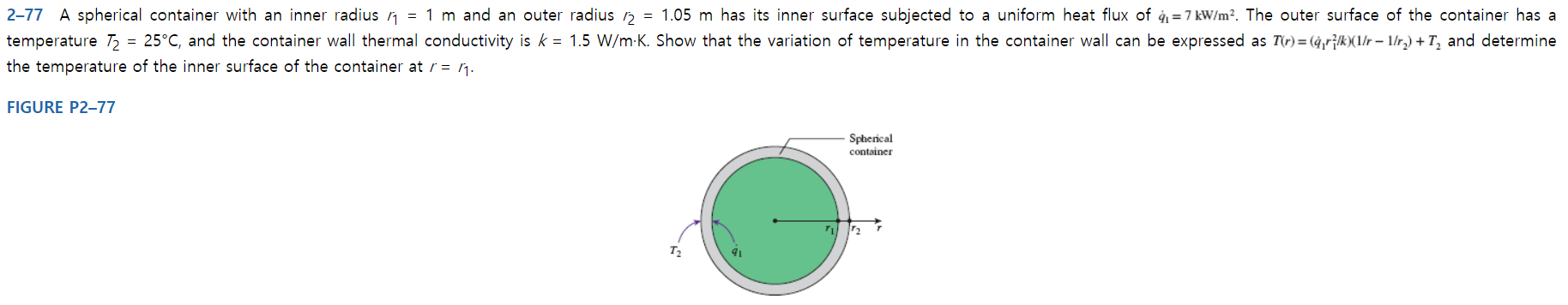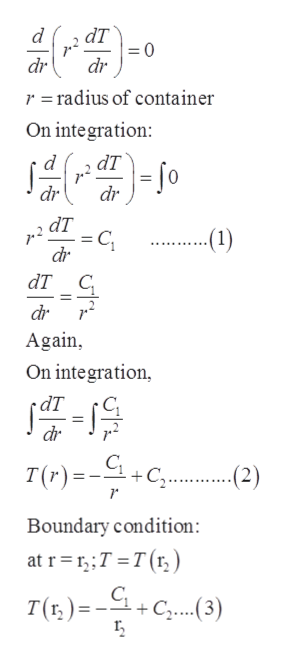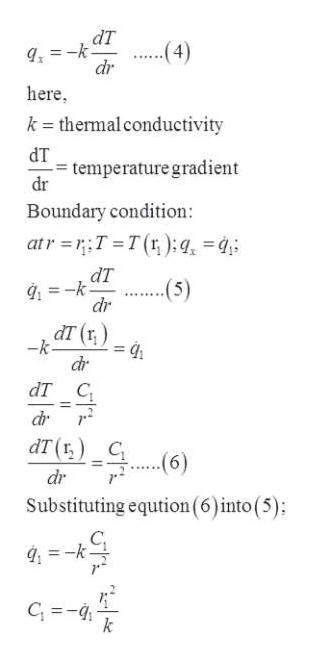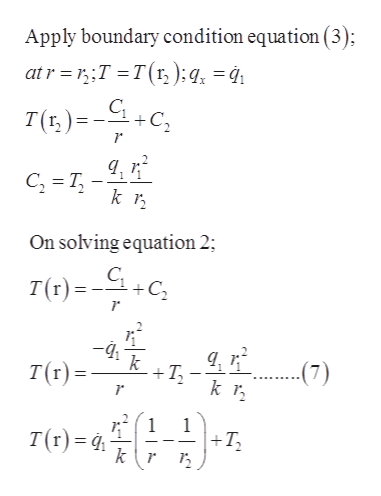= 1.05 m has its inner surface subjected to a uniform heat flux of q1=7 kW/m2. The outer surface of the container has a2-77 A spherical container with an inner radius n = 1 m and an outer radius25°C, and the container wall thermal conductivity is k 1.5 W/m K. Show that the variation of temperature in the container wall can be expressed as T(r)= (4,r^k(1r 1/r.) + T2 and determinetemperature T2the temperature of the inner surface of the container at r = ^FIGURE P2-77SphericalcontainerT2

Question

A spherical container with an inner radius r1 = 1 m and an outer radius r2 = 1.05 m has its inner surface subjected to a uniform heat flux of q1=7kw/m^2. The outer surface of the container has a temperature T2 = 25°C, and the container wall thermal conductivity is k = 1.5 W/m·K. Show that the variation of temperature in the container wall can be expressed as  and determine the temperature of the inner surface of the container at r = r1.help_outlineImage Transcriptionclose= 1.05 m has its inner surface subjected to a uniform heat flux of q1=7 kW/m2. The outer surface of the container has a 2-77 A spherical container with an inner radius n = 1 m and an outer radius 25°C, and the container wall thermal conductivity is k 1.5 W/m K. Show that the variation of temperature in the container wall can be expressed as T(r)= (4,r^k(1r 1/r.) + T2 and determine temperature T2 the temperature of the inner surface of the container at r = ^ FIGURE P2-77 Spherical container T2 fullscreen
Step 1

Using differential equation for heat conduction,help_outlineImage TranscriptioncloseаT =0 dr d dr r = radius of container On integration d dT dr dr dT C dr --(1) t 111 1 111 ат с dr Again On integration dr T(r)=C 2) Boundary condition at rT T(r) C, +C..3) fullscreen
Step 2

Using Fourier’s law in radial r-direction,help_outlineImage TranscriptionclosedT k dr (4) here k - thermal conductivity dT temperature gradient dr Boundary condition: atr=T T(r );q, dT (5) dr dт (t) -k dr dT C 11 dr dT(rC (6) dr Substituting eqution (6)into (5) g-k fullscreen
Step 3

Calculation:

&n...help_outlineImage TranscriptioncloseApply boundary condition equation (3); atr T T(r, );q = 4 T()C C T k On solving equation 2; T(r)=C -4i T k T(r)= (7) 1 1 T(r) |+T3 k\r fullscreen

Want to see the full answer?

See Solution

Want to see this answer and more?

Our solutions are written by experts, many with advanced degrees, and available 24/7

See Solution
Tagged in

Heat Transfer